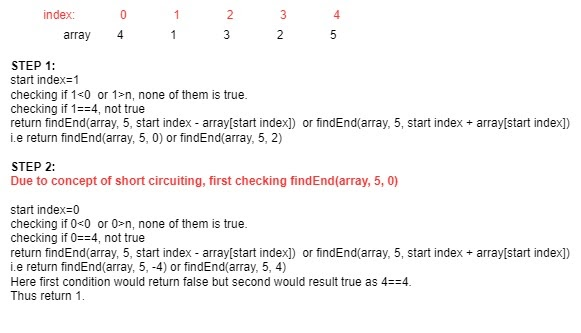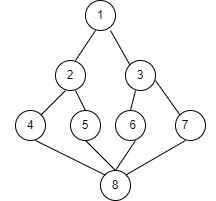# Check if the end of the Array can be reached from a given position.

## Introduction

In this blog, we will discuss a recursion problem asked frequently in Interviews.

The problem is to check if the end of the Array can be reached from a given position.We are given N numbers in an array and a starting position, and we are asked to check if the end of the Array can be reached from a given position on a condition that we can only move either (current index + array[current index]) or (current index - array[current index]).

Example: Say 5 elements in an array given are: 4, 1, 3, 2, 5

And the start index given is 1.

We have to check if we can reach the end of the array starting from position 1, following all the given conditions.

## Recursive Approach

The approach to Check if the end of the Array can be reached from a given position would be straightforward if we follow the recursive method.
Time Complexity = O(n)

If the index is negative or greater than the array size, then we definitely can't reach the end, so return false.

If we have reached the last index, we can reach the end and return true.

Use recursion to change position and check if now we can get the end of the array.

Solving the above given example,### PseudoCode

Algorithm

___________________________________________________________________

procedure ifEnd(int a[], int n, int start_idx):

___________________________________________________________________

1.  If start_idx<0 or start_idx>n

return false;

2.   If start_idx==n-1

return true;

3.   return ifEnd(a,n,start_idx-a[start_idx]) or ifEnd(a,n,start_idx+a[start_idx].

4.   Declare main function, give user input and return answer.

end procedure

___________________________________________________________________

### Implementation in C++

``````#include <iostream>
using namespace std;

bool ifEnd(int a[], int n, int start_idx){

//if index is negative or greater than size of array then definitely we can't reach the end.
if(start_idx<0 || start_idx>n){
return false;
}

//if we have reached the last index, this means that it is possible to reach the end.
if(start_idx==n-1){
return true;
}

//using recursion to change position and checking if we can reach the end of the array.
return ifEnd(a,n,start_idx-a[start_idx]) || ifEnd(a,n,start_idx+a[start_idx]);
}

int main(){
int n;
cin>>n;
int a[n];

for(int i=0; i<n; i++){
cin>>a[i];
}
int src;
cin>>src;
bool result=ifEnd(a,n,src);
cout<<result;

}``````

Output

``````Sample Input:
5
4 1 3 2 5
1
Sample Output:
Yes``````

#### Complexity Analysis

Time Complexity: O(n)

Analysing Time Complexity:

In the worst-case, all elements are traversed once in the recursive call stack.

∴n.

Space complexity: O(n).

## BFS Approach

This problem can also be solved using the BFS approach:

• The graph G and array visited[] are global, and visited is initialized to 0.
• Then  u=v, visited[v]=1
• Repeat, for all vertices w, adjacent to u do, if visited[w]=0, add w to queue and visited[w]=1
• If the queue is empty, return and delete the next element u from the queue.

• BFS(G,n)
• For i=1 to n do visited[i]=0, for i=1 to n, do if(visited[i]=0)then BFS(i)visiting order: 2,3,4,5,6,7,8

Visited queue: 1 0 0 0 0 0 0 0

u=1, w={2,3}

q: 2 3 remove 2

u=2, w={1,4,5}

q: 3 4 5 remove 3

u=3, w={1,6,7}

q: 4 5 6 7 remove 4

u=4, w={2,8}

q: 5 6 7 8 remove 5

u=5, w={2,8}

u=6, w={3,8}

u=7, w={3,8}

u=8, w={4,5,6,7}

So same algorithm would be used to check if the end of the Array can be reached from a given position with the condition given that we can only move either (current index + array[current index]) or (current index - array[current index])

Till now, I assume you must have got the basic idea of what has been asked in the problem statement. So, I strongly recommend you first give it a try.

try here

Please have a look at the algorithm, and then again, you must give it a try.

### PseudoCode

___________________________________________________________________

procedure solve(int a[], int n, int start_idx):

___________________________________________________________________

1.  queue is required in bfs approach because of its FIFO approach. queue<int> q;

2.  bool visited[n] = { false };

bool reached = false;

3.  q.push(start);

4.  Until q becomes empty:

5.  int temp = q.front();

q.pop();

6.   if visited[temp] == true: continue

7.    visited[temp] = true;

8.    if (temp == n - 1)

reached = true;

break;

9.    if (temp + arr[temp] < n)

q.push(temp + arr[temp]);

10.   if (temp - arr[temp] >= 0)

q.push(temp - arr[temp]);

11.  Then, if (reached == true)

Print yes else print no.

end procedure

___________________________________________________________________

### Implementation in C++

``````#include <bits/stdc++.h>
using namespace std;

void solve(int arr[], int n, int start)
{

// queue is required in bfs approach because of its FIFO approach
//write algorithm for bfs.
queue<int> q;

// At first all nodes are unvisited, and we have not reach the end.
bool visited[n] = { false };
bool reached = false;

// Inserting first element in queue
q.push(start);

// Until queue becomes empty
while (!q.empty()) {

// Get the top element and delete it
int temp = q.front();
q.pop();

// If already visited ignore that index
if (visited[temp] == true)
continue;

visited[temp] = true;
//when we have reached the end of the array
if (temp == n - 1) {
reached = true;
break;
}

// as we can move only temp + arr[temp] or temp - arr[temp], so inserting that in the queue.
if (temp + arr[temp] < n) {
q.push(temp + arr[temp]);
}

if (temp - arr[temp] >= 0) {
q.push(temp - arr[temp]);
}
}

// If we can reach the end of the array,print yes else print no.
if (reached == true) {
cout << "Yes";
}
else {
cout << "No";
}
}

// Driver Code
int main()
{
int n,s;
cin>>n;
int arr[n];
for(int i=0; i<n; i++){
cin>>arr[i];
}
cin>>s;
solve(arr, n, s);
return 0;
}``````

Output

``````Sample Input:
5
4 1 3 2 5
1
Sample Output:
Yes``````

#### Complexity Analysis

Time Complexity: O(n).

Analysing Time Complexity:

In the worst-case, all elements are traversed once.

∴n.

Space complexity: O(n).

1. When to use the breadth-first search method?
Answer) Whenever we need to search part of the tree as a solution to the problem where depth can vary, we use the breadth-first approach.
2. What is recursion?
3. When to use recursion?
Answer)When we can break the problem into smaller parts, we can use recursion. It also helps in reducing the complexity sometimes.

## Key Takeaways

This article taught us how to Check if the end of the Array can be reached from a given position by approaching the problem using recursion. We discussed its implementation using illustrations, pseudocode, and then proper code.

We hope you could take away critical techniques like analyzing problems by walking over the execution of the examples and finding out the recursive pattern followed.

Now, we recommend you practice problem sets based on recursion to master your fundamentals. You can get a wide range of questions similar to this on CodeStudio